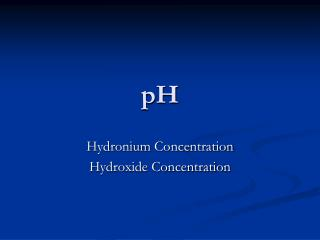DownloadDownload PresentationHydronium Concentration Hydroxide Concentration

# Hydronium Concentration Hydroxide Concentration

Télécharger la présentation## Hydronium Concentration Hydroxide Concentration

- - - - - - - - - - - - - - - - - - - - - - - - - - - E N D - - - - - - - - - - - - - - - - - - - - - - - - - - -
##### Presentation Transcript

1. pH Hydronium Concentration Hydroxide Concentration

2. Acid vs Base • When acidity and basicity are exactly balanced such that the numbers of H3O+ and OH− ions are equal, we say that the solution is neutral. Neutral = H3O+ = OH- • Pure water is neutral because it contains equal amounts of the two ions.

3. pH • In 1909, Danish chemist SørenSørensen proposed using the negative of the power of 10 (that is, the negative logarithm) of [H3O+] as the index of basicity and acidity. • He called this measure the pH. The letters p and H represent power of hydrogen. • pH can be calculated by the following mathematical equation: pH = −log [H3O+]

4. Calculating pH from [H3O+] • Because of the negative sign, as the hydronium ion concentration increases, the pH will decrease. • A solution of pH 0 is very acidic. • A solution of pH 14 is very alkaline. • A solution of pH 7 is neutral.

5. pH

6. Calculating pH • The pH equation may be rearranged to calculate the hydronium ion concentration from the pH. [H3O+] = 10−pH • Because pH is related to powers of 10, a change in one pH unit corresponds to a tenfold change in the concentrations of the hydroxide and hydronium ions.

7. The Self-Ionization Constant of Water • An equilibrium-constant expression relates the concentrations of species involved in an equilibrium. • The relationship for the water equilibrium is simply [H3O+][OH−] = Keq. • This equilibrium constant, called the self-ionization constant is so important that it has a special symbol, Kw.

8. The Self-Ionization Constant of WaterKw [H3O+][OH−] = Kw Kw = (1.00 × 10−7)(1.00 × 10−7) = 1.00 × 10−14 • The product of these two ion concentrations is always a constant. • Anything that increases one of the ion concentrations decreases the other. • If you know one of the ion concentrations, you can calculate the other.

9. Common pH values

10. pH indicators • Certain dyes, known as indicators, turn different colors in solutions of different pH. • An indicator a compound that can reversibly change color depending on the pH of the solution or other chemical change • pH Meters • A pH meter is an electronic instrument equipped with a probe that can be dipped into a solution. • The probe has two electrodes, one of which is sensitive to the hydronium ion.

11. Text pg 544 Calculating pH for an Acidic or Basic Solution Sample Problem B What is the pH of (a) a 0.000 10 M solution of HNO3, a strong acid, and (b) a 0.0136 M solution of KOH, a strong base?

12. Chapter 15 Section2 Acidity, Basicity, and pH Calculating pH for an Acidic or Basic Solution,continued • Sample Problem B Solution • Concentration of HNO3 solution = 0.000 10 M = 1.0 × 10−4 M; pH = ? • (b) Concentration of KOH solution = 0.0136 M = 1.36 × 10−2 M; pH = ? • Kw= 1.00 × 10−14

13. Chapter 15 Section2 Acidity, Basicity, and pH Calculating pH for an Acidic or Basic Solution, continued Sample Problem B Solution (a)pH = −log [H3O+] = −log (1.0 × 10−4) = −(–4.00) = 4.00 (b) pH = −log [H3O+] = −log (7.35 × 10−13) = −(−12.13)= 12.13

14. Text pg 545 Calculating [H3O+] and [OH−] from pH Sample Problem C What are the concentrations of the hydronium and hydroxide ions in a sample of rain that has a pH of 5.05?

15. Chapter 15 Section2 Acidity, Basicity, and pH Calculating [H3O+] and [OH−] from pH, continued Sample Problem C Solution pH = 5.05 [H3O+] = ? Kw= 1.00 × 10−14 [OH−] = ? (a) [H3O+] = 10−pH = 10−5.05 = 8.9 × 10−6 M

16. Assignment • Practice Problems • Text pg 541 # 1-5 • Text pg 544 # 1-4 • Text pg 545 # 1-4